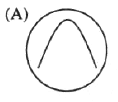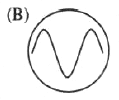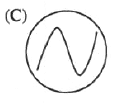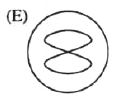GR 8677927796770177 | # Login | Register

GR9277 #17
Problem
 GREPhysics.NET Official Solution Alternate Solutions
\prob{17}
The outputs of two electrical oscillators are compared to an oscilloscope screen. The oscilloscope spot is initialy at the center of hte screen. Oscillator Y is connected to the vertical terminals of the oscilloscope and oscillator X to the horizontal terminals. Which of the following patterns could appear on the oscilloscope screen, if the frequency of oscillator Y is twice that of oscillator X.

1.2.3.4.5.Lab Methods}Oscilloscope

This problem can be solved by elimination. Since one is given two waves, one with twice the frequency of the other, one can approximate the superposed wave (which shows up on the oscilloscope) as .

The summed wave no longer looks like a sine wave. Instead, it looks like a series of larger amplitude humps alternating with regions of smaller amplitudes.

However, since one is not supplied with a graphing calculator on the test, one can qualitatively eliminate the other choices based on the equation above. It is obviously not choices (D) and (E) since the superposition is still a one-to-one function. It isn't choice (C) or (B) since those are just sin waves (cosine waves are just off by a phase), and one knows that the superposed wave would look more complicated than that. Thus, one arrives at choice (A), which is a zoomed-in-view of the superposition above.Alternate Solutions
 behnamn902013-10-14 17:09:46 The graph on the oscilloscope is a parametric plot with the parameter being angular frequency ranging from zero to 2pi. This is called a "Lissajous figure" on an oscilloscope used in X-Y mode. The question asks which one is possible? If we have y=-cos(2w) and x=-cos(w) you get (A). If you have y=sin(2w) and x=sin(w) you get (E) rotated 90 degrees so (E) isn't possible. Go on: http://www.wolframalpha.com/input/?i=parametric+plot+y%3D-cos%282t%29+and+x%3D-cos%28t%29 The correct one is plotted in the link.Reply to this commentkstephe62012-11-04 18:37:52 NO MATH HERE!! This is old skool Electrical Engineer knowledge. We have a 1:2 frequency ratio. This means the pattern has ONE "hump" in it. **Extra info below**** (google phase shift chart to get a better idea of what I mean here) All patterns for the 1:2 ratio look like a 3D wire frame of a Pringle's potato chip or horse saddle rotated about the vertical. (Again, ONE HUMP) The answer here happens to be the simplest corresponding to a phase shift of 45 degrees which is a side view of the Pringle's potato chip where only the front have is visible and the back half of the chip is eclipsed. Again, google the chart. You'll see.rnrnReply to this commentnyuko2009-11-04 07:10:44 To solve this problem, all I did was to follow the trace and imagine a projection on X and Y. Then you see in (A), Y completes a round trip while X completes half a round trip. So the frequency in Y is twice that of X. Done! (Sorry, I meant to label this as "user solution" yet didn't know it would be reset after preview)Reply to this commentjburkart2007-11-01 00:20:57 If we first assume , , then we can solve: , so , which then reduces to . This is not any of the figures listed, because this problem is extremely unreasonable. However, if we assume instead that and , then it resolves into , choice (A).Reply to this commentsenatez2006-10-20 09:56:06 I think it is best to think of it as a vector function which traces out a curve: f(t) = sin(t)i + sin(2t)j This can immediately elliminate D and E which would be a vector of the form of an ellipse: f(t) = cos(t)i + sin(t)jReply to this commentytsejam2006-10-17 11:16:56 The explanation given above is wrong. The oscilloscope is in X-Y mode. So the answer has to be a Lissajous figure. All the options are Lissajous figures for various frequency ratios. A) omega_Y = 2* omega_X B)omega_Y = 4* omega_X C)omega_Y = 3* omega_X D)omega_Y = omega_X E)omega_Y = 1/2 *omega_XReply to this commentg7d
2014-10-20 18:56:31
This demonstration should make things clearer. http://demonstrations.wolfram.com/VirtualOscilloscope/salticus
2014-10-13 11:19:44
It's a mistake to assume both oscillators are in phase.
What says the oscillators were "switched on" at the same time?

In general, not just .

Using :

produces an infinity shape,
produces a parabola opening upward or a "U" shape,
produces that infinity shape again,
and gives a parabola opening downward or a "frown."

Instead of calculating all that out, I like kstephe6 or nyuko approached it.
kstephe6: imagine the outline of a saddle (i.e. pringles potato chip shape) rotating around the z-axis.
nyuko: check the ratios, and pick the one that fits.behnamn90
2013-10-14 17:09:46
The graph on the oscilloscope is a parametric plot with the parameter being angular frequency ranging from zero to 2pi. This is called a "Lissajous figure" on an oscilloscope used in X-Y mode. The question asks which one is possible? If we have y=-cos(2w) and x=-cos(w) you get (A). If you have y=sin(2w) and x=sin(w) you get (E) rotated 90 degrees so (E) isn't possible. Go on:
http://www.wolframalpha.com/input/?i=parametric+plot+y%3D-cos%282t%29+and+x%3D-cos%28t%29

The correct one is plotted in the link.
 behnamn902013-10-14 17:28:53 The shape you get is also very dependant on the phase difference; see: http://en.wikipedia.org/wiki/Lissajous_curve Anyone know how to quickly get the answer?kstephe6
2012-11-04 18:37:52
NO MATH HERE!!

This is old skool Electrical Engineer knowledge.

We have a 1:2 frequency ratio. This means the pattern has ONE "hump" in it.

**Extra info below****

(google phase shift chart to get a better idea of what I mean here)

All patterns for the 1:2 ratio look like a 3D wire frame of a Pringle's potato chip or horse saddle rotated about the vertical. (Again, ONE HUMP)

The answer here happens to be the simplest corresponding to a phase shift of 45 degrees which is a side view of the Pringle's potato chip where only the front have is visible and the back half of the chip is eclipsed.

Again, google the chart. You'll see.rnrn
 kstephe62012-11-05 05:06:59 In fact, E could be answer IF it were rotated 90 degrees in the plane of the page
 memyselmineni2014-11-19 10:17:46 I agree! No maths at all. Only frequency ratio is needed! Get help from this book, it nails the physics GRE! http://www.amazon.com/Physics-Mathematica-Jude-Ndubuisi-Onicha/dp/1499691920/ref=sr_1_fkmr1_1?s=books&ie=UTF8&qid=1401627044&sr=1-1-fkmr1&keywords=physics+mathematica++2nd+edition+by+onicha+judealanalan
2012-09-11 16:11:22
Something is terribly wrong here! If answer E is correct when X is twice the frequency of Y, then it should be the same but rotated by 90 degrees when Y is twice the frequency of X. You are just swapping the axis when the frequencies are swapped.

That means none of the answers are correct!niux
2009-11-06 10:39:39
ETS were nonsense in this answer. The condition that spot is initially at the center of the screen: IT IS THIS INITIAL CONDITION, that inmediatly discard (A), (B) and (D). NO MATTER WHAT THE RATIO OF FREQUENCY IS.
 niux2009-11-06 10:45:36 My last line is wrong, answer shuould not be (E) beacuse ratio of frequency is 1:2 (y:x) and not (2:1). Anyway, as neither (C) satisfies this condition. I Repeat: ETS were non sense in this one
 neon372010-11-10 11:36:45 have you never used an oscilloscope? It refreshes everyone once in a while depending on what you set. The cursor being at the center means that the oscilloscope is calibrated or rather adjusted in such a way. This shapes that appear are the oscillations about the center and not spatially shifted.nyuko
2009-11-04 07:10:44
To solve this problem, all I did was to follow the trace and imagine a projection on X and Y. Then you see in (A), Y completes a round trip while X completes half a round trip. So the frequency in Y is twice that of X. Done!

(Sorry, I meant to label this as "user solution" yet didn't know it would be reset after preview)nyuko
2009-11-04 07:09:36
To solve this problem, all I did was to follow the trace and imagine a projection on X and Y. Then you see in (A), Y completes a round trip while X completes half a round trip. So the frequency in Y is twice that of X. Done!nyuko
2009-10-30 09:50:21
I never remember a single expression for this kind of problem. I even didn't know the trace has a fancy name.

To solve this problem, all I did was to follow the trace and imagine a projection on X and Y. Then you see in (A), Y completes a round trip while X completes half a round trip. So the frequency in Y is twice that of X. Done!chrisfizzix
2008-10-06 11:19:20
This is a totally dick question.

Just to be constructive about it and all.
 GREmania2008-10-12 06:08:07 yeah, it is. Hahajburkart
2007-11-01 00:20:57
If we first assume , , then we can solve: , so , which then reduces to . This is not any of the figures listed, because this problem is extremely unreasonable. However, if we assume instead that and , then it resolves into , choice (A).jburkart
2007-11-01 00:20:39
If we first assume , , then we can solve: , so , which then reduces to . This is not any of the figures listed, because this problem is extremely unreasonable. However, if we assume instead that and , then it resolves into , choice (A).dcb
2007-09-16 14:51:46
While I initially thought it was E (a Lissajous curve), writing a simple example sheds some light.

Lets take a time interval from 0 to 8 (the scale is arbitrary for an easy integer example).
- In this interval, X will go from 0 to 4 and back to 0 (one oscillation).
- Y, with twice the frequency, will go from 0 to 4 to 0 to 4 to 0.

Simply, (using a linear example):

T X Y
0 0 0
1 1 2
2 2 4
3 3 2
4 4 0 <--(at this point, X has gone through half a period,
5 3 2 Y has gone through a full period)
6 2 4
7 1 2
8 0 0

Plot X vs Y, It a simple triangular looking hump, similar to A. It is linear because I made the input waves linear for simplicity. However, it does not have to be.

 danksci2008-09-08 14:29:09 According to the question shouldnt 0 strart at the center of the screen?
 taatliberty2011-10-11 14:54:51 Yeah. Plus, Y should go negative as it continues through the oscillation.kwooley
2007-04-06 19:08:43
http://ibiblio.org/e-notes/Lis/Lissa.htmhamood
2007-04-01 16:23:46
y = 1,0,-1,0,1,0,-1,0,1 (period 0f 4)
x=4,3,2,1,0,1,2,3,4 (period of 8)
The plotted graph looks likes A....SonOfOle
2006-11-02 21:27:32
x123. Check the link you supplied again. They give a Lissajous curve that looks exactly like (A) on that page where the ratio of the frequencies is 1:2.senatez
2006-10-20 09:56:06
I think it is best to think of it as a vector function which traces out a curve:

f(t) = sin(t)i + sin(2t)j

This can immediately elliminate D and E which would be a vector of the form of an ellipse:

f(t) = cos(t)i + sin(t)jsenatez
2006-10-20 09:52:29ytsejam
2006-10-17 11:16:56
The explanation given above is wrong. The oscilloscope is in X-Y mode. So the answer has to be a Lissajous figure. All the options are

Lissajous figures for various frequency ratios.

A) omega_Y = 2* omega_X

B)omega_Y = 4* omega_X

C)omega_Y = 3* omega_X

D)omega_Y = omega_X

E)omega_Y = 1/2 *omega_X
 D8less2007-10-02 17:30:06 Thank you, I was waiting for someone to say this.
 k942008-04-07 18:56:58 This is a the right answer http://ibiblio.org/e-notes/Lis/Lissa.htm
 Poop Loops2008-10-05 13:41:47 This is by far the easiest way to look at it.
 kroner2009-11-04 23:35:31 To be a nitpicky, (B) is actually just a nonsensical image. Notice at both extremes of X, Y seems to turn around at zero rather than reaching -1.
 crossno2010-11-12 18:03:01 I agree, this is the correct way to do it. except both B and C are omega_y=3*omega_x.....the only difference is the phase the answer is Ax123
2006-10-17 08:56:46
I think the answer is (E), because it's a Lissajous curve.

http://en.wikipedia.org/wiki/Lissajous_curve
 europa2006-11-01 22:11:01 ETS says it is A but only 10 percent scored this answer correctly so go figure...
 ducksquark2007-11-02 05:28:41 It should be a Lissajous figure all right. But (E) shows the figure for the wrong freq. ratio. (X being twice the freq of Y -- see wikipedia article -- the example figure given there is (E) turned on is side.)
 k942008-04-07 18:57:18 http://ibiblio.org/e-notes/Lis/Lissa.htm
 taatliberty2011-10-11 15:01:25 Europe, where did you get the answer list?It's a mistake to assume both oscillators are in phase. What says the oscillators were "switched on" at the same time? In general, not just . Using : produces an infinity shape, produces a parabola opening upward or a "U" shape, produces that infinity shape again, and gives a parabola opening downward or a "frown." Instead of calculating all that out, I like kstephe6 or nyuko approached it. kstephe6: imagine the outline of a saddle (i.e. pringles potato chip shape) rotating around the z-axis. nyuko: check the ratios, and pick the one that fits. (see their comments for details.)LaTeX syntax supported through dollar sign wrappers $, ex.,$\alpha^2_0$produces . type this... to get...$\int_0^\infty\partial\Rightarrow\ddot{x},\dot{x}\sqrt{z}\langle my \rangle\left( abacadabra \right)_{me}\vec{E}\frac{a}{b}\$